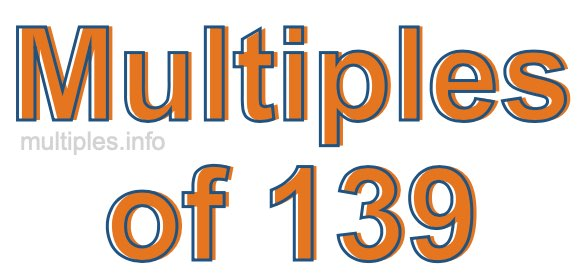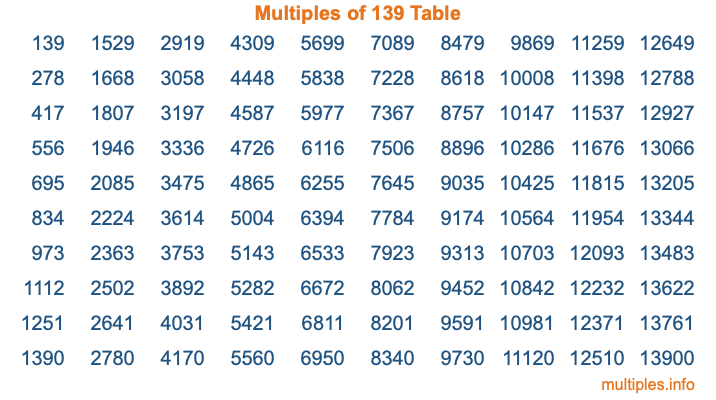Multiples of 139Welcome to the Multiples of 139 page. Here we will first teach you everything you will ever need to know about the multiples of 139, and then give you a study guide summary of everything we taught you to make sure you remember it all. Use this page to look up facts and learn information about the multiples of 139. This page will make you a multiples of one hundred thirty-nine expert!

Definition of Multiples of 139
Multiples of 139 are all the numbers that when divided by 139 equal an integer. Each of the multiples of 139 are called a multiple. A multiple of 139 is created by multiplying 139 by an integer.

Therefore, to create a list of multiples of 139, you start with 1 multiplied by 139, then 2 multiplied by 139, then 3 multiplied by 139, and so on for as long as you want. Thus, the list of the first five multiples of 139 is 139, 278, 417, 556, and 695. To see a larger list of multiples of 139, see the printable image of Multiples of 139 further down on this page. We also have a category where you can choose any nth multiple of 139.

Multiples of 139 Checker
The Multiples of 139 Checker below checks to see if any number of your choice is a multiple of 139. In other words, it checks to see if there is any number (integer) that when multiplied by 139 will equal your number. To do that, we divide your number by 139. If the the quotient is an integer, then your number is a multiple of 139.

Is  a multiple of 139?

Least Common Multiple of 139 and ...
A Least Common Multiple (LCM) is the lowest multiple that two or more numbers have in common. This is also called the smallest common multiple or lowest common multiple and is useful to know when you are adding our subtracting fractions. Enter one or more numbers below (139 is already entered) to find the LCM.

Check out our LCM Calculator if you need more details about the Least Common Multiple or if you need the LCM for different numbers for adding and subtraction fractions.

nth Multiple of 139
As we stated above, 139 is the first multiple of 139, 278 is the second multiple of 139, 417 is the third multiple of 139, and so on. Enter a number below to find the nth multiple of 139.

th multiple of 139

Multiples of 139 vs Factors of 139
139 is a multiple of 139 and a factor of 139, but that is where the similarities end. All postive multiples of 139 are 139 or greater than 139. All positive factors of 139 are 139 or less than 139.

Below is the beginning list of multiples of 139 and the factors of 139 so you can compare:

Multiples of 139: 139, 278, 417, 556, 695, etc.

Factors of 139: 1, 139

As you can see, the multiples of 139 are all the numbers that you can divide by 139 to get a whole number. The factors of 139, on the other hand, are all the whole numbers that you can multiply by another whole number to get 139.

It's also interesting to note that if a number (x) is a factor of 139, then 139 will also be a multiple of that number (x).

Multiples of 139 vs Divisors of 139
The divisors of 139 are all the integers that 139 can be divided by evenly. Below is a list of the divisors of 139.

Divisors of 139: 1, 139

The interesting thing to note here is that if you take any multiple of 139 and divide it by a divisor of 139, you will see that the quotient is an integer.

Multiples of 139 Table
Below is an image of the first 100 multiples of 139 in a table. The table is in chronological order, column by column. The first column has the first ten multiples of 139, the second column has the next ten multiples of 139, and so on.The Multiples of 139 Table is also referred to as the 139 Times Table or Times Table of 139. You are welcome to print out our table for your studies.

Negative Multiples of 139
Although not often discussed or needed in math, it is worth mentioning that you can make a list of negative multiples of 139 by multiplying 139 by -1, then by -2, then by -3, and so on, to get the following list of negative multiples of 139:

-139, -278, -417, -556, -695, etc.

Multiples of 139 Summary
Below is a summary of important Multiples of 139 facts that we have discussed on this page. To retain the knowledge on this page, we recommend that you read through the summary and explain to yourself or a study partner why they hold true.

There are an infinite number of multiples of 139.

A multiple of 139 divided by 139 will equal a whole number.

139 divided by a factor of 139 equals a divisor of 139.

The nth multiple of 139 is n times 139.

The largest factor of 139 is equal to the first positive multiple of 139.

139 is a multiple of every factor of 139.

139 is a multiple of 139.

A multiple of 139 divided by a divisor of 139 equals an integer.

139 divided by a divisor of 139 equals a factor of 139.

Any integer times 139 will equal a multiple of 139.

Multiples of a Number
Here you can get the multiples of another number, all with the same attention to detail as we did for multiples of 139 on this page.

Multiples of
Multiples of 140
Did you find our page about multiples of one hundred thirty-nine educational? Do you want more knowledge? Check out the multiples of the next number on our list!